×

Search anything:

# 3D Geometry: Mathematics for Computational Geometry

#### computational geometry List of Mathematical AlgorithmsGet this book -> Problems on Array: For Interviews and Competitive Programming

In this article, we have covered the Mathematics of 3D Geometry for Computational Geometry. This involve the exploration of different 3D shapes along with area and volume of each shape.

• Introduction
• 3D definition
• 3D space
• 3D shape calculations
• Overview

# Introduction

We will be covering the basis of 3D geometry. This will expand on the concepts we have introduced in the previous articles and give a wider basis to geometry as a whole.

# 3D definition

In comparison to 2D, 3D includes an extra dimension, depth. This can be represented in coordinates with the letter Z. We can say a tuple of 3 numbers (x, y, z) can be interpreted as 3 dimensional Euclidean space.

# 3D Space

Axis and planes differ in 3D from 2D due to the need to account for the height of an object, its z coordinate.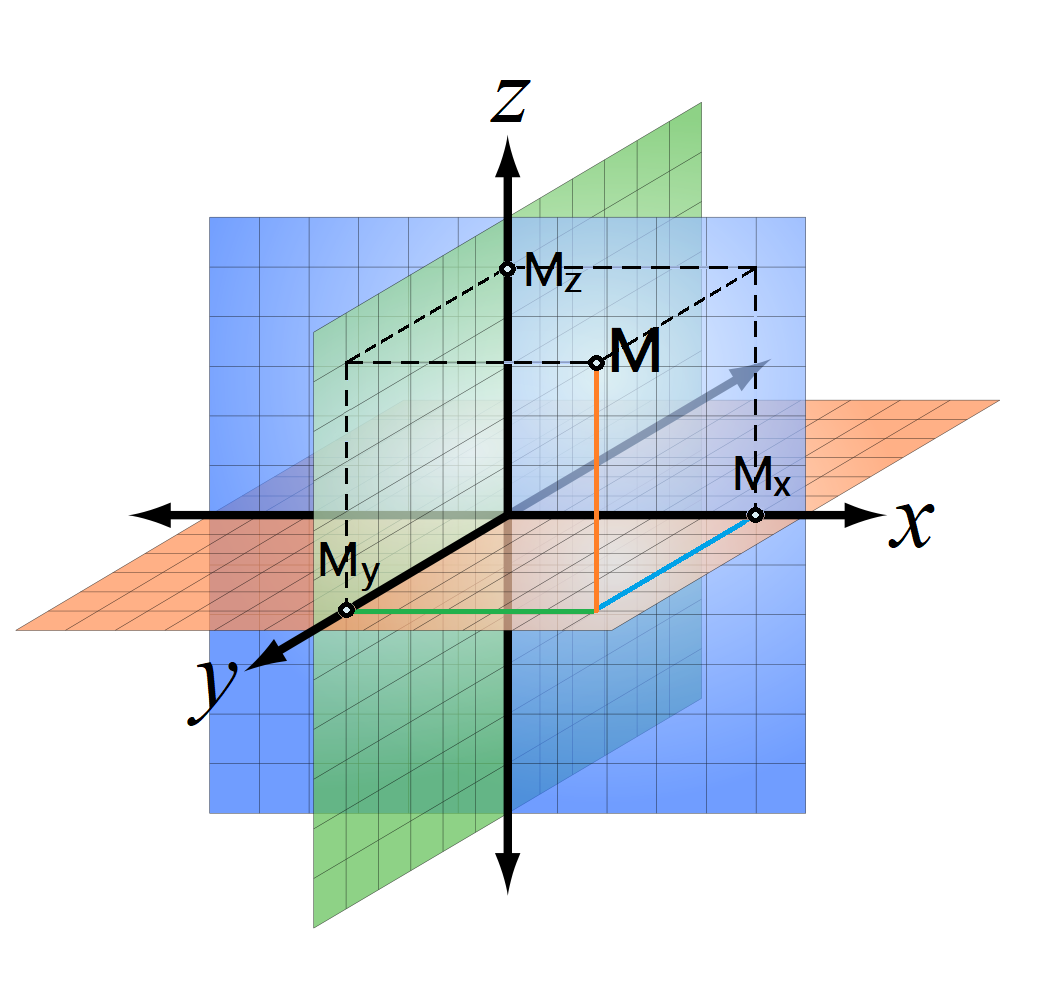As you can see above, the 3D coordinate axis are made from three intersecting planes. The intersection of all three is called the origin, these planes split the 3D space into 8 octants.

Let there be a perpendicular line to M going downwards, from this point we extend perpendicular lines to My and Mx, we can say that the length of these lines are the coordinates of the points x, y and z. From this we can deduce that if a coordinate is located on an axis its coordinates will be (x,0,0) for the x axis, (0,y,0) for y and (0,0,z) for z. These can be combined for example a point with only X and Z value could be written as (x,0,z) and so on.

## Distance between 2 points in 3D

To calculate the distance between 2 points in 3D, we need to adjust the Euclidean formula used for 2D. It is much the same however with the addition of the Z coordinate.

``````Points: (x1,y1,z1) and (x2,y2,z2)

d = √(x2-x1)^2+(y2-y1)^2+(z2-z1)^2
``````

## Equation of line in 3D space

To find the equation of a line in 3D space, we can follow certain steps, it is different from 2D since we now need to account for the Z coordinate.

• Find the direction ratios of the points by finding the difference between each of the coordinates of the two points (eg. x2-x1, y2-y1, z2-z1)
• Choose any of the two points
• Write the required equation to pass through the points: L: (x-x1)/l=(y-y1)/m=(z-z1)

With (x,y,z) being position coordinates of any point lying on the straight line.

# 3D Shape calculations

There are generally 2 basic calculations we can carry out on 3D shapes to find its properties, surface area and volume.

Surface area is the amount of material that needs to be used to cover the shape. It is essentially, the area around the outside of a 3D shape, without accounting for the space inside it.

The volume of shape can be thought of as how much space it takes up.

We will describe how to find the volume and surface area of useful 3D shapes using various formulas.

## Cuboid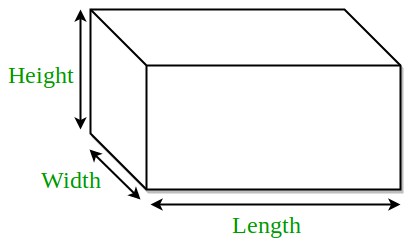### Surface Area

A cuboid is made up of 6 rectangles which make up the faces therefore to find the surface area, we need to find the area of all 6 of these rectangles

``````SA = A(rect1 + rect2...+ rect6)
SA = (l*h) + (l*w) + (l*h) + (l*w) + (w*h) + (w*h)
Simplified -> SA = 2(lh + lw + wh)

where l is length, h is height and w is width
``````

### Volume

The simplest formula is probably the volume of a cuboid, we have to multiply each different type of side in order to get the total volume.

``````Vol = Length * Width * Height
``````

## Cube

### Surface Area

A cube is similar to a cuboid however with all sides equal in length, since we know this, we can deduce: length = height = width

``````From SA of cuboid -> SA = 2(lh + lw + wh)
We know l = h = w
Therefore SA of cube = 2(3l^2)
SA = 6l^2

where l is length
``````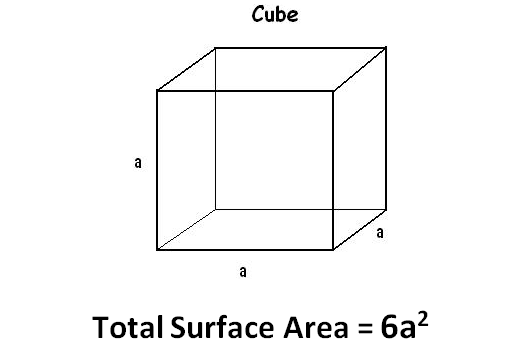### Volume

We know a cube is a similar shape to a cuboid, however with equal sides. We can derive its volume as such:

``````Vol = Length * Width * Height
L = W = H
Let a = one of the three side types
Therefore, Vol = a^3
``````## Cylinder

### Surface Area

A cylindrical shape will be easier to work with if we decompose it, we can see it is formed with a rectangle and two circles.

``````Circumference of cylinder = 2πr
Therefore SA = 2πrh
Total SA = SA of cylinder + Area of base
SA = 2πrh + 2πr^2 or 2πr(r+h)
``````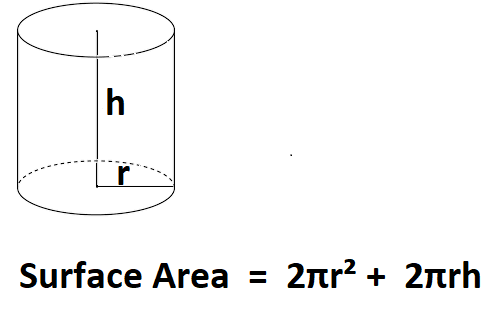### Volume

If we unroll a cylinder, generally we will be able to find a base and height. Therefore we can say:

``````Base area (circle) = πr^2
Height = h
Therefore, Vol = πr^2h
``````## Sphere

### Surface Area

We can split a sphere into 4 circles in order to get the surface area

``````Area of circle = πr^2
Therefore SA of sphere = 4πr^2
``````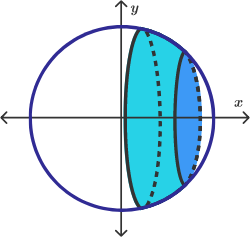### Volume

The volume of a sphere formula was created by Archimedes in around 250BC. Originally it may have been hard to derive (since calculus was not invented yet), but it is fairly easy with modern techniques.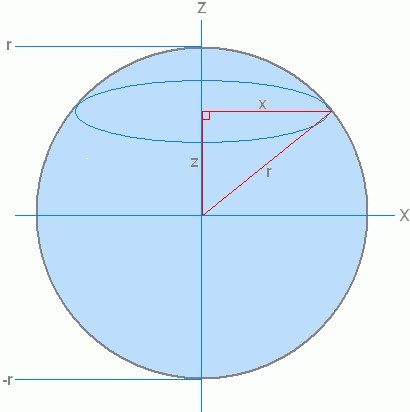Take the sphere above, if we apply Pythagorous' formula we can get:
x^2 + z^2 = r^2

To find x we shall rearrange to get: x = √r^2-z^2
This gives us the radius, as we know the area of a circle is πr^2 therefore we can times pi by the square of this to get the area.

A = π(√r^2-z^2)^2 or A = π(r^2 - z^2)

To get the volume of the sphere, we will have to find the area of all of these circles which make it up. We can see from the diagram that the top of the circle is r and bottom is -r.

If we integrate the area we can get the volume, using the limits of the top and bottom of the circle r and -r.

-rr π(r^2-z^2)dz
= π((2/3r^3) - (-2/3r^3))
= 4/3πr^3

Leaving us with the final volume of 4/3πr^3

## Cone

### Surface Area

The surface area of a cone can again solved by unravelling the shape. A cone is two radii of a sector brought together. The surface area of a cone is the sum of its base and the lateral side surface area.

``````SA = 1/2*l*2πr
SA = πrl
SA = πrl + πr^2
``````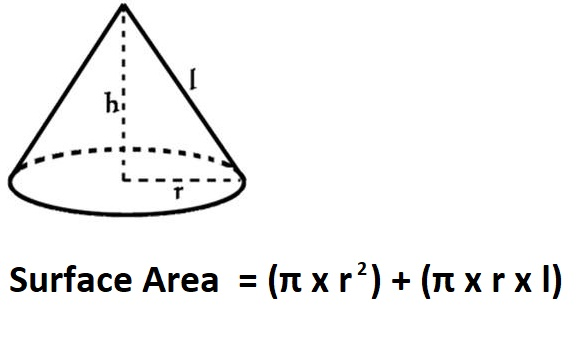### Volume

We can find the volume of cone by taking the base area, multiplying it by height. It is much similar to the volume of a cylinder however since a cone is two radii of the same sector, we need to take 1/3 since it will not take the same space as a cylinder, leaving us with the equation:

``````Vol = 1/3πr^2h
``````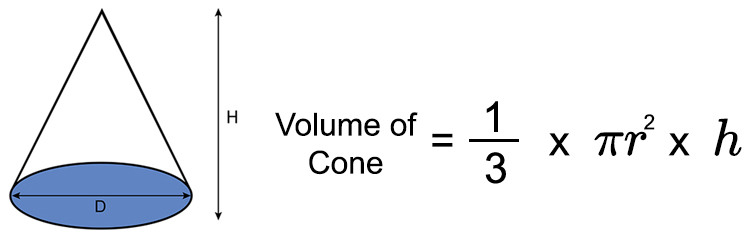## Hemisphere

### Surface Area

A hemisphere is a sphere divided in half by a plane. It is essentially half a sphere.

``````We know that SA of sphere = 4πr^2
SA of lateral hemisphere = 2πr^2
We also need to add the base for the total SA, therefore
SA = 3πr^2
``````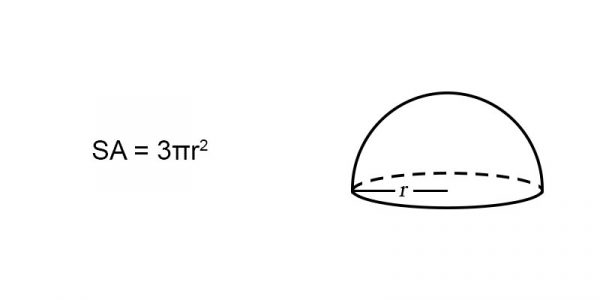### Volume

The volume of a hemisphere is simply half that of a sphere.

``````We derived that the volume of a sphere was 4/3πr^3,
meaning that the volume of a hemisphere = 2/3πr^3
``````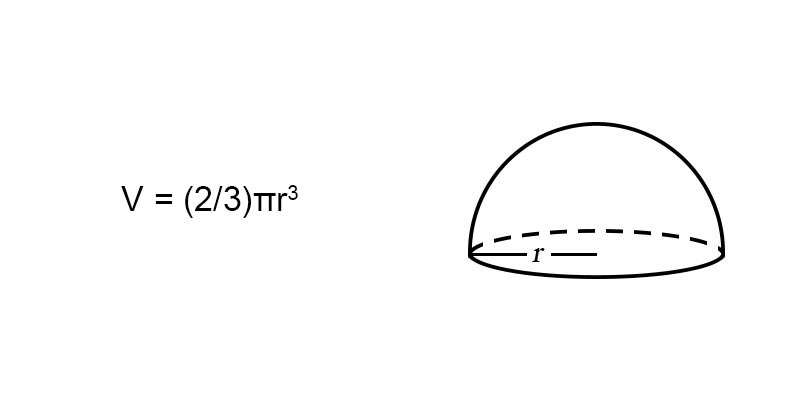## Prism

### Surface Area

We can split a prism into numerous shapes in order to derive its surface area. For our particular prism (Triangular base), this will be two rectangles and three triangles (two sides, one base).

``````SA = 2(Area of triangles) + 2(Area of side rectangles) + (Area of base rectangle)
SA = (b*h)+2(s*l)+(l*b)
``````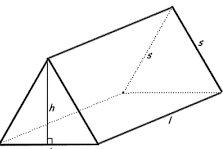### Volume

A prism will be similar to a rectangle to find the area, we need to take the area of the base, multiplied by the height and length of the prism.

``````Vol = 1/2b*h*l
``````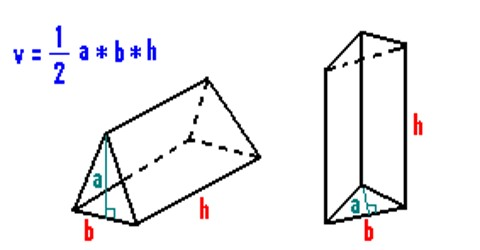# Overview

In this article at OpenGenus, we have introduced 3D space and shapes, describing various aspects such as planes and axis, along with adjustments needed for 3D calculation of things such as distance between points. As well as detail numerous useful formulas for surface area and volume for many essential shapes.